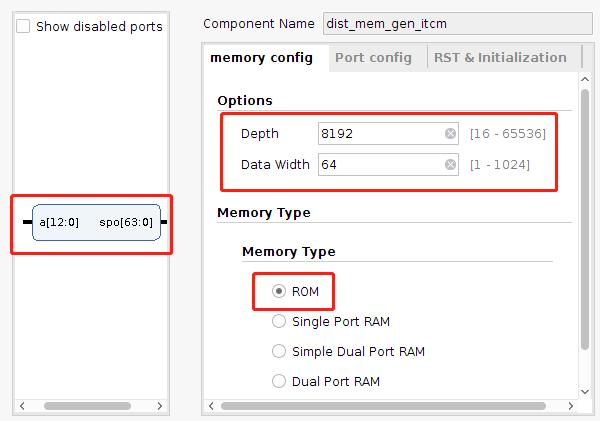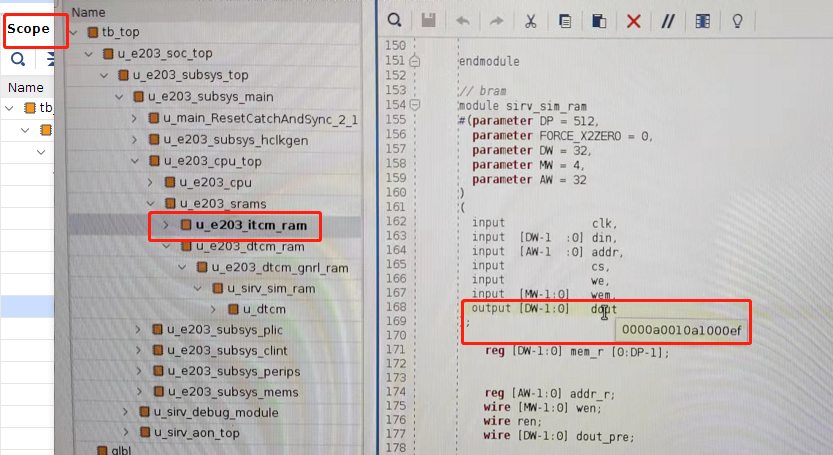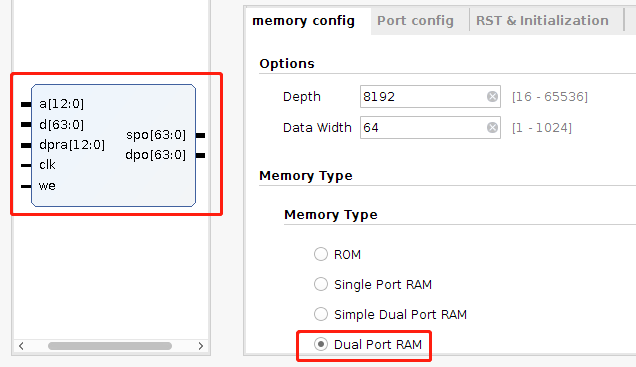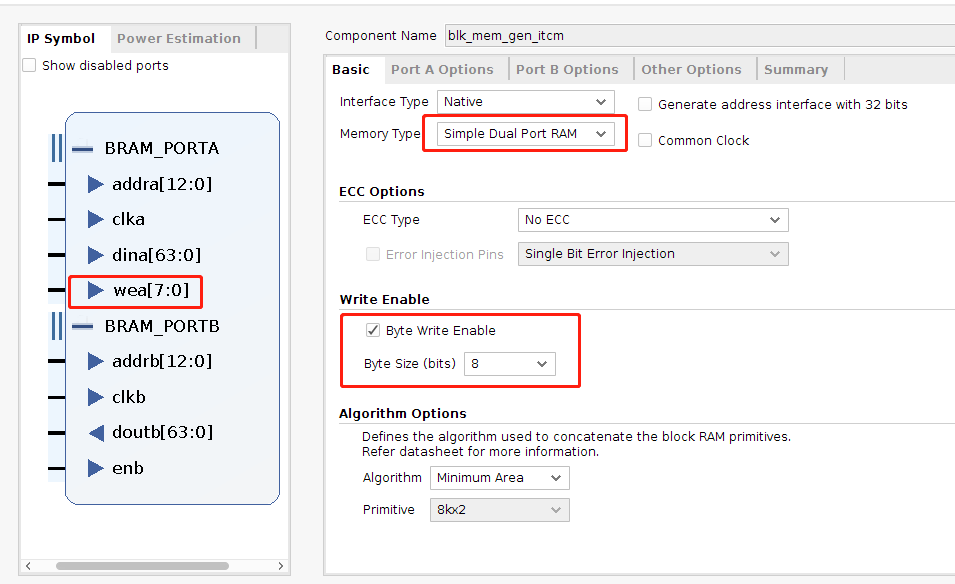# Hummingbirdv2 E203 移植之固化itcm

## 一、Flash不够

• ilm： 直接将代码写入指令存储器(itcm)，速度最快，但掉电会消失
• flash：先保存到mcu flash，然后再拷贝到itcm上执行，掉电不消失
• flashxip：直接保存到mcu flash，并读flash运行，速度较慢

## 二、修改itcm

E203的指令存储器是通过寄存器实现的，如果我们把它修改成只读存储器ROM，然后预先读入.coe文件，那么就可以和E203一起烧入flash，这样一上电就可以运行代码。

//读部分逻辑
reg [DW-1:0] mem_r [0:DP-1];  //DW=32,DP=8192
always @(posedge clk)
begin
if (ren) begin
end
end
assign dout_pre = mem_r[addr_r];#python hello.verilog
import argparse
parser = argparse.ArgumentParser()

args = parser.parse_args()
total_len = 0
str_16 = []
with open(args.file,"r",encoding="utf-8") as f:
for line in f:
if(line=="@"):
temp = eval("0x"+line.strip("\n")[1:])
for i in range(temp - total_len):
str_16.append("00")
total_len += 1
else:
temp = line.strip("\n").split(" ")
for i in range(len(temp)):
str_16.append(temp[i])
total_len +=1
for i in range(8-total_len % 8):
str_16.append("00")

row = int(total_len//8)+1
for i in range(row):
file_data += str_16[i*8+7]+ str_16[i*8+6]+str_16[i*8+5]+str_16[i*8+4]\
+str_16[i*8+3]+str_16[i*8+2]+str_16[i*8+1]+str_16[i*8+0]+",\n"
with open("out.coe","w",encoding= "utf-8") as f:
f.write(file_data)


## 三、恶梦debugtip: 仿真到bug出现的时刻点，然后可以在代码中查看此刻各信号的数值，大大加快速度。

## 四、能不能加入写功能？

itcm的写功能主要用于调试，借助其SDK，通过Jtag口将代码直接传入itcm，方便debug，否则每次修改代码都需要重新烧一遍板子，非常费时费力。module sirv_sim_ram_itcm
#(parameter DP = 512,
parameter FORCE_X2ZERO = 0,
parameter DW = 32,
parameter MW = 4,
parameter AW = 32
)
(
input             clk,
input  [DW-1  :0] din,
input             cs,
input             we,
input  [MW-1:0]   wem,
output [DW-1:0]   dout
);

wire [MW-1:0] wen;
wire ren;

wire [DW-1:0] dout_pre,spo,din_temp,wen_ext;
assign din_temp = (wen_ext & din) | (~wen_ext &spo);  //下一时刻更新数据
assign wen = ({MW{cs & we}} & wem);
assign wen_ext={ {8{wen}},{8{wen}},{8{wen}},{8{wen}},{8{wen}},{8{wen}},{8{wen}},{8{wen}}};

dist_mem_gen_rewr u_rewr(
.d(din_temp),   //写数据
.clk(clk),
.we(we),       //读使能
.spo(spo),    //写地址的当前数据，需要结合wen和din得到下一时刻的更新数据din_temp
.dpo(dout_pre)   //读数据
);

assign ren = cs & (~we);
always @(posedge clk)
begin
if (ren) begin
end
end
endmodule

## 五、能不能用BRAM？module sirv_sim_ram
#(parameter DP = 512,
parameter FORCE_X2ZERO = 0,
parameter DW = 32,
parameter MW = 4,
parameter AW = 32
)
(
input             clk,
input  [DW-1  :0] din,
input             cs,
input             we,
input  [MW-1:0]   wem,
output [DW-1:0]   dout
);
wire [MW-1:0] wen;
wire ren;
wire [DW-1:0] dout_pre;

assign ren = cs & (~we);
assign wen = ({MW{cs & we}} & wem);

blk_mem_gen_itcm u_dtcm(
.clka(clk),
.dina(din),
.wea(wen),
.clkb(clk),
.doutb(dout_pre),
.enb(ren)
);

endmodule

• itcm的位宽是64bit，长度8192；dtcm的位宽是32bit，长度是16384，这一点在e203_define.v和config.v里面有说明，添加IP的时候要注意这两个不同点。
• Basic 勾选 Write Enable，Byte Size 选8bits
• Port A Options ，Enable Port Type 选择“Always Enabled”
• PortB Options，不要选 Primitives Output Register
• Summary 确认 PortB Latency为1 Cycles